## Example Questions

### Example Question #1 : How To Find The Diagonal Of A Cube

The surface area of a cube is 486 units.  What is the distance of its diagonal (e.g. from its front-left-bottom corner to its rear-right-top corner)?

9√(3)

81

None of the others

9

9√(2)

9√(3)

Explanation:

First, we must ascertain the length of each side.  Based on our initial data, we know that the 6 faces of the cube will have a surface area of 6x2. This yields the equation:

6x2 = 486, which simplifies to: x2 = 81; x = 9.

Therefore, each side has a length of 9.  Imagine the cube is centered on the origin.  This means its "front-left-bottom corner" will be at (–4.5, –4.5, 4.5) and its "rear-right-top corner" will be at (4.5, 4.5, –4.5).  To find the distance between these, we use the three-dimensional distance formula:

d = √((x1 – x2)2 + (y1 – y2)2 + (z1 – z2)2)

For our data, this will be:

√( (–4.5 – 4.5)2 + (–4.5 – 4.5)2 + (4.5 + 4.5)2) =

√( (–9)2 + (–9)2 + (9)2) = √(81 + 81 + 81) = √(243) =

√(3 * 81) = √(3) * √(81) = 9√(3)

### Example Question #2 : How To Find The Diagonal Of A Cube

You have a rectangular box with dimensions 6 inches by 6 inches by 8 inches. What is the length of the shortest distance between two non-adjacent corners of the box?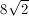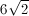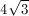Explanation:

The shortest length between any two non-adjacent corners will be the diagonal of the smallest face of the rectangular box. The smallest face of the rectangular box is a six-inch by six-inch square. The diagonal of a six-inch square is.

### Example Question #3 : How To Find The Diagonal Of A Cube

What is the length of the diagonal of a cube with side lengths of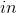each?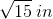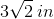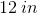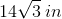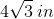Explanation:

The diagonal length of a cube is found by a form of the distance formula that is akin to the Pythagorean Theorem, though with an additional dimension added to it. It is: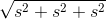, or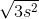, or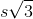Now, if the the value ofis, we get simply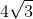### Example Question #4 : How To Find The Diagonal Of A Cube

What is the length of the diagonal of a cube that has a surface area of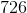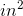?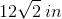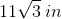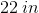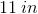Explanation:

To begin, the best thing to do is to find the length of a side of the cube. This is done using the formula for the surface area of a cube. Recall that a cube is made up ofsquares. Therefore, its surface area is: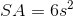, whereis the length of a side.

Therefore, for our data, we have: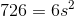Solving for, we get: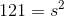This means that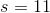Now, the diagonal length of a cube is found by a form of the distance formula that is akin to the Pythagorean Theorem, though with an additional dimension added to it. It is:, or, orNow, if the the value ofis, we get simply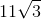Tired of practice problems?

Try live online GRE Math prep today.